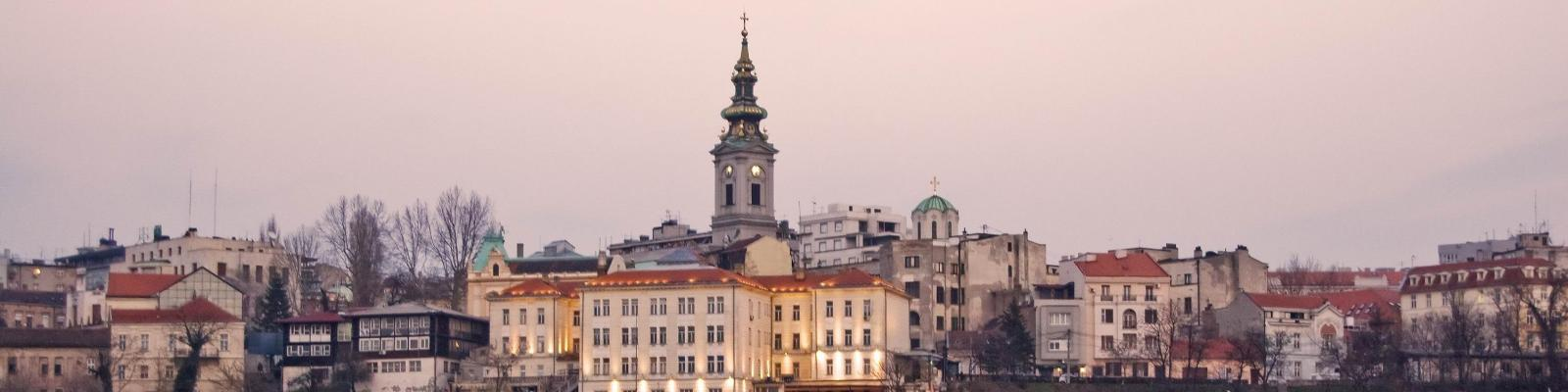QUANTUM STRUCTURE of SPACETIME and GRAVITY 2016## Harold Steinacker: Fuzzy spaces and applications

The lectures on fuzzy spaces will be structured as follows:

Lecture 1 with provide the basics of fuzzy spaces (i.e. quantized embedded symplectic spaces). This will cover the 2-dimensional fuzzy sphere, the fuzzy torus, fuzzy CP^n, and more generally quantized coadjoint orbits.

Lecture 2 will discuss some further structures on fuzzy spaces, in particular coherent states, string states & the separation of UV / IR regimes, and Laplace- and Dirac operators. Furthermore, the 4-dimensional fuzzy sphere will be briefly introduced.

In Lecture 3 some physical applications will be discussed, in particular noncommutative field theory and gauge theory as described by matrix models. The phenomenon of UV/IR mixing will be explained. If time permits & depending on the interests, either fuzzy extra dimensions in Yang-Mills gauge theory or emergent gravity on fuzzy S^4 will be briefly discussed.